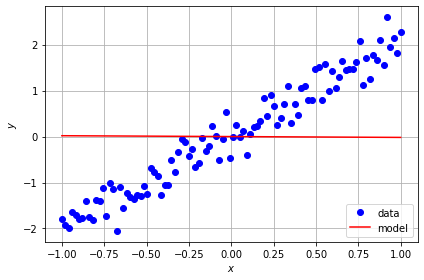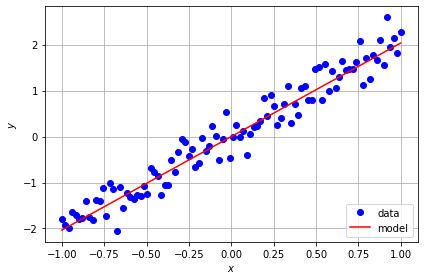# Exercise 4.2 - Solution¶

## Linear regression¶

In this task we will design and train a linear model using Keras.

1. Complete the implemetation of the LinearLayer
2. Define a meaningful objective
3. Implement gradient descent and train the linear model for 80 epochs.
In :
import tensorflow as tf
from tensorflow import keras
import numpy as np
import matplotlib.pyplot as plt

layers = keras.layers


### Simulation of data¶

Let's first simulate some noisy data

In :
np.random.seed(1904)
x = np.float32(np.linspace(-1, 1, 100)[:,np.newaxis])
y = np.float32(2 * x[:,0] + 0.3 * np.random.randn(100))
print("x.shape:", x.shape)
print("y.shape:", y.shape)

x.shape: (100, 1)
y.shape: (100,)


### Implement linear model¶

Now, we have to design a linear layer that maps from the input $x$ to the output $y$ using a single adaptive weight $w$:

$$y = w \cdot x$$

Complete the implementation of the LinearLayer by adding the linear transformation in the call function.

In :
class LinearLayer(layers.Layer):

def __init__(self, units=1, input_dim=1):  # when intializing the layer the weights have to be initialized
super(LinearLayer, self).__init__()
w_init = tf.random_normal_initializer()
self.w = tf.Variable(initial_value=w_init(shape=(input_dim, units), dtype="float32"),
trainable=True)

def call(self, inputs):  # when calling the layer the linear transformation has to be performed
return tf.matmul(inputs, self.w)


Build a model using the implemented layer.

In :
model = keras.models.Sequential()

In :
model.build((None, 1))
print(model.summary())

Model: "sequential"
_________________________________________________________________
Layer (type)                 Output Shape              Param #
=================================================================
linear_layer (LinearLayer)   (None, 1)                 1
=================================================================
Total params: 1
Trainable params: 1
Non-trainable params: 0
_________________________________________________________________
None


### Performance before the training¶

Plot data and model before the training

In :
y_pred = model(x)

fig, ax = plt.subplots(1)
ax.plot(x, y, 'bo', label='data')
ax.plot(x, y_pred, 'r-', label='model')
ax.set(xlabel='$x$', ylabel='$y$')
ax.grid()
ax.legend(loc='lower right')
plt.tight_layout()### Task 2: Define the objective function¶

Define a meaningful objective here (regression task).
Note that you can use tf.reduce_mean() to average your loss estimate over the full data set (100 points).

In :
def loss(x, y):
return tf.reduce_mean((tf.squeeze(x)-tf.squeeze(y))**2)


'Train' the linear model for 80 epochs (or iterations) with a meaningful learning rate and implement gradient descent.
Hint: you can access the adaptive parameters using model.trainable_weights and perform $w' \rightarrow w-z$ using w.assign_sub(z)

In :
epochs = 80  # number of epochs
lr = 0.1  # learning rate

for epoch in range(epochs):

output = model(x, training=True)
# Compute loss value
loss_value = loss(tf.convert_to_tensor(y), output)

print("Current loss at epoch %d: %.4f" % (epoch, float(loss_value)))

Current loss at epoch 0: 1.5236
Current loss at epoch 1: 1.3338
Current loss at epoch 2: 1.1689
Current loss at epoch 3: 1.0257
Current loss at epoch 4: 0.9014
Current loss at epoch 5: 0.7933
Current loss at epoch 6: 0.6995
Current loss at epoch 7: 0.6180
Current loss at epoch 8: 0.5472
Current loss at epoch 9: 0.4857
Current loss at epoch 10: 0.4323
Current loss at epoch 11: 0.3859
Current loss at epoch 12: 0.3456
Current loss at epoch 13: 0.3106
Current loss at epoch 14: 0.2802
Current loss at epoch 15: 0.2538
Current loss at epoch 16: 0.2308
Current loss at epoch 17: 0.2109
Current loss at epoch 18: 0.1936
Current loss at epoch 19: 0.1786
Current loss at epoch 20: 0.1655
Current loss at epoch 21: 0.1542
Current loss at epoch 22: 0.1443
Current loss at epoch 23: 0.1357
Current loss at epoch 24: 0.1283
Current loss at epoch 25: 0.1219
Current loss at epoch 26: 0.1163
Current loss at epoch 27: 0.1114
Current loss at epoch 28: 0.1072
Current loss at epoch 29: 0.1035
Current loss at epoch 30: 0.1003
Current loss at epoch 31: 0.0975
Current loss at epoch 32: 0.0951
Current loss at epoch 33: 0.0930
Current loss at epoch 34: 0.0912
Current loss at epoch 35: 0.0896
Current loss at epoch 36: 0.0882
Current loss at epoch 37: 0.0871
Current loss at epoch 38: 0.0860
Current loss at epoch 39: 0.0851
Current loss at epoch 40: 0.0843
Current loss at epoch 41: 0.0837
Current loss at epoch 42: 0.0831
Current loss at epoch 43: 0.0826
Current loss at epoch 44: 0.0821
Current loss at epoch 45: 0.0817
Current loss at epoch 46: 0.0814
Current loss at epoch 47: 0.0811
Current loss at epoch 48: 0.0809
Current loss at epoch 49: 0.0806
Current loss at epoch 50: 0.0804
Current loss at epoch 51: 0.0803
Current loss at epoch 52: 0.0801
Current loss at epoch 53: 0.0800
Current loss at epoch 54: 0.0799
Current loss at epoch 55: 0.0798
Current loss at epoch 56: 0.0797
Current loss at epoch 57: 0.0797
Current loss at epoch 58: 0.0796
Current loss at epoch 59: 0.0795
Current loss at epoch 60: 0.0795
Current loss at epoch 61: 0.0795
Current loss at epoch 62: 0.0794
Current loss at epoch 63: 0.0794
Current loss at epoch 64: 0.0794
Current loss at epoch 65: 0.0793
Current loss at epoch 66: 0.0793
Current loss at epoch 67: 0.0793
Current loss at epoch 68: 0.0793
Current loss at epoch 69: 0.0793
Current loss at epoch 70: 0.0793
Current loss at epoch 71: 0.0792
Current loss at epoch 72: 0.0792
Current loss at epoch 73: 0.0792
Current loss at epoch 74: 0.0792
Current loss at epoch 75: 0.0792
Current loss at epoch 76: 0.0792
Current loss at epoch 77: 0.0792
Current loss at epoch 78: 0.0792
Current loss at epoch 79: 0.0792


### Performance of the fitted model¶

Plot data and model after the training

In :
fig, ax = plt.subplots(1)

y_pred = model(x)

ax.plot(x, y, 'bo', label='data')
ax.plot(x, y_pred, 'r-', label='model')
ax.set(xlabel='$x$', ylabel='$y$')
ax.grid()
ax.legend(loc='lower right')
plt.tight_layout()